# Common potential and loss of energy on sharing charges.

• 1
•
•
•
•
•
•
•
1
Share

## COMMON POTENTIAL AND LOSS OF ENERGY ON SHARING CHARGES

### COMMON POTENTIAL

When two capacitors of different potential are connected by a conducting wire, then the charges flows from capacitor at higher potential to the capacitor at lower potential. This flow of charges continues till their potential become equal, this equal potential is called common potential.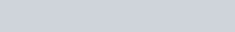Where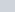and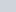are capacitance of the two capacitors and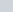and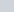are the potential respectively.
So the common potential is given as-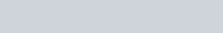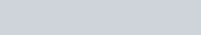From this equation, we can say that, charge lost by one capacitor is equal to charge gained by other capacitor.
But here you have to keep in mind that, this is not true for potential. i.e, potential lost by one capacitor is not equal to the potential gained by other. Because their capacitance are different.

### LOSS OF ENERGY ON SHARING OF CHARGES

When two charged capacitors are connected to each other, they start sharing their charges till they acquire a common potential. On sharing charges there is always some loss of energy. However, total charge of the system remains conserved.

Let’s consider two capacitors having capacitanceandand their potential isand. Then, the total energy stored in the capacitor before the two capacitors are connected is-

(1)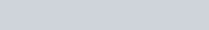When the two capacitors are connected together, the total charge on the capacitor is-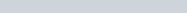And the total capacitance of the two capacitors are-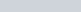Now, the total energy stored in the capacitor after they are connected is given as-

(2)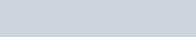After subtracting equ(1) and equ(2), we get-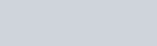We see that, this is a positive quantity. Since U – U’ is positive, so we conclude that there is always some loss of energy, when two charged capacitors are connected together, in the form of heat radiation die to electric current while charging.